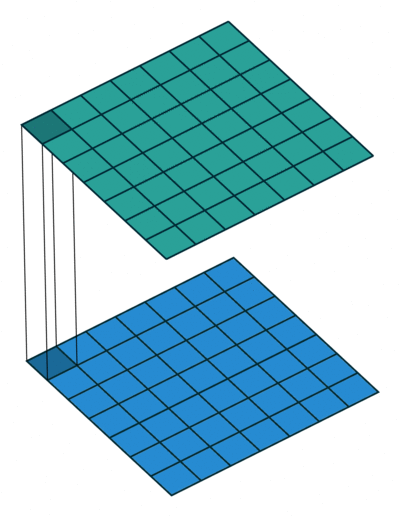# Different Convolutions

### Pointwise Convolution or 1x1 convolution

It is a 1x1 convolution kernel. This helps in acquiring pixel by pixel level feature extraction. But the main purpose is,1. When the number channels in the previous layers needs to be shrinked , then we use 1x1 convolution. This reduces the problem of computational cost.
2. For all the features learned from the previous layer, 1x1 convolution indroduces more nonlinearity ( through activation function ). This inturn increases the network’s nonlinearity with the previous layers.

Andrew Ng’s coursera video on 1x1 convolution

### Dilated ConvolutionA kernel with spaces in between each cell is called dilation. 1-Dilated kernel has 1 cell spaces around pixels. 1-Dilated kernels have the capacity to extract higher spatial information from an effective receptive field. Dilated kernels can be used along with regular kernels. Since, a 0-Dilated kernel is a regular kernel .

Dilated convolution are used for applications like semantic segmentation with one label per pixel , image super resolution , denoising etc.

$$Dilated \ Kernel = \begin{bmatrix} 1 & 0 & 1 & 0 & 1 \\ 0 & 0& 0&0&0\\ 1 & 0 & 1 & 0 & 1 \\ 0 & 0& 0&0&0\\ 1 & 0 & 1 & 0 & 1 \\ \end{bmatrix}$$ CS231n Notes on Dilated convolution

Dilated Convolution Blog Post

This paper infers that the architecture assumes the fact that dilated convolution increases the receptive field exponentially through the network.

### Transposed convolution layer / Deconvolution

From the paper Deconvolution Networks

Convolutional networks are a bottom-up approach where the input signal is subjected to multiple layers of convolutions, non-linearities and sub-sampling. By contrast, each layer in our Deconvolutional Network is top-down; it seeks to generate the input signal by a sum over convolutions of the feature maps (as opposed to the input) with learned filters.